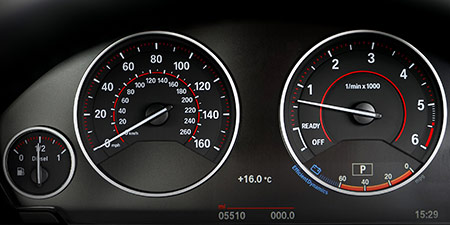# Miles per hour (mph) to Yards per second (yd/s)

Units of speed ﹣ Converter Miles per hour to Yards per second

Here you can convert the Speed unit Miles per hour into the unit Yards per second and vice versa you can convert Yards per second into Miles per hour. By clicking the "Swap units" icon, you will always obtain the desired conversion in the calculation result, i.e., mph to yd/s or yd/s to mph. With the following calculator you can also calculate any other Speed unit.

## Info about "Miles per hour"

The unit of length known as a "mile" and it should not be confused with the nautical mile and very commonly used in the Anglo-American countries. Therefore, the metric system is defined in miles (instead of kilometers) per time unit. For example, if a vehicle travels at a speed of 100 miles per hour (100 mph), it will move around 160 km per hour in the metric system.

The units are defined as follows: An object moving at 1 "mph" per hour will cover a distance of one mile. Therefore, 1 mile is equivalent to 1.609344 kilometers.

## Info about "Yards per second"

The length unit "Yard" is commonly used in Anglo-American countries. For example, speeds are represented by yard per unit of time. The walking speed of a pedestrian is around one yard per second (y/s).

Definition: An object moving at 1 "yard per second" per second will cover a distance of one yard. Therefore, 1 yard is equivalent to 0.9144 meters (3 feet).

## Basis for conversion Miles per hour (mph) to Yards per second (yd/s) and vice versa

The abbreviation for the "Speed unit Miles per hour" is mph. The abbreviation for the "Speed unit Yards per second" is yd/s.

## Formula for the conversion of Miles per hour (mph) to Yards per second (yd/s) and vice versa

The calculation from Miles per hour to Yards per second shall be made using the following conversion formula:

Conversion formula Miles per hour to Yards per second

Determine the number of Yards per second from Miles per hour

Miles per hour × 0.48888888869219

## Formula for the conversion of Yards per second (yd/s) to Miles per hour (mph)

The calculation from Yards per second to Miles per hour shall be made using the following conversion formula:

Conversion formula Yards per second to Miles per hour

Determine the number of Miles per hour from Yards per second

Yards per second × 2.0454545462775

## Overview table : How many Miles per hour are in a Yards per second ?

Miles per hour mph ⇒ Yards per second yd/s
0.01 mph  are  0.00488 yd/s
0.02 mph  are  0.00977 yd/s
0.03 mph  are  0.01466 yd/s
0.04 mph  are  0.01955 yd/s
0.05 mph  are  0.02444 yd/s
0.06 mph  are  0.02933 yd/s
0.07 mph  are  0.03422 yd/s
0.08 mph  are  0.03911 yd/s
0.09 mph  are  0.04400 yd/s
0.10 mph  are  0.04888 yd/s
0.20 mph  are  0.09777 yd/s
0.30 mph  are  0.14666 yd/s
0.40 mph  are  0.19555 yd/s
0.50 mph  are  0.24444 yd/s
0.60 mph  are  0.29333 yd/s
0.70 mph  are  0.34222 yd/s
0.80 mph  are  0.39111 yd/s
0.90 mph  are  0.44000 yd/s
1 mph  corresponds to  0.48888 yd/s
2 mph  are  0.97777 yd/s
3 mph  are  1.46666 yd/s
4 mph  are  1.95555 yd/s
5 mph  are  2.44444 yd/s
6 mph  are  2.93333 yd/s
7 mph  are  3.42222 yd/s
8 mph  are  3.91111 yd/s
9 mph  are  4.40000 yd/s
10 mph  are  4.88888 yd/s
20 mph  are  9.77777 yd/s
30 mph  are  14.66666 yd/s
40 mph  are  19.55555 yd/s
50 mph  are  24.44444 yd/s
60 mph  are  29.33333 yd/s
70 mph  are  34.22222 yd/s
80 mph  are  39.11111 yd/s
90 mph  are  44 yd/s
100 mph  are  48.88888 yd/s
200 mph  are  97.77777 yd/s
300 mph  are  146.66666 yd/s
400 mph  are  195.55555 yd/s
500 mph  are  244.44444 yd/s
600 mph  are  293.33333 yd/s
700 mph  are  342.22222 yd/s
800 mph  are  391.11111 yd/s
900 mph  are  440 yd/s
1 000 mph  are  488.88888 yd/s

## Overview table : How many Yards per second are in a Miles per hour ?

Yards per second yd/s ⇒ Miles per hour mph
0.01 yd/s  are  0.02045 mph
0.02 yd/s  are  0.04090 mph
0.03 yd/s  are  0.06136 mph
0.04 yd/s  are  0.08181 mph
0.05 yd/s  are  0.10227 mph
0.06 yd/s  are  0.12272 mph
0.07 yd/s  are  0.14318 mph
0.08 yd/s  are  0.16363 mph
0.09 yd/s  are  0.18409 mph
0.10 yd/s  are  0.20454 mph
0.20 yd/s  are  0.40909 mph
0.30 yd/s  are  0.61363 mph
0.40 yd/s  are  0.81818 mph
0.50 yd/s  are  1.02272 mph
0.60 yd/s  are  1.22727 mph
0.70 yd/s  are  1.43181 mph
0.80 yd/s  are  1.63636 mph
0.90 yd/s  are  1.84090 mph
1 yd/s  corresponds to  2.04545 mph
2 yd/s  are  4.09090 mph
3 yd/s  are  6.13636 mph
4 yd/s  are  8.18181 mph
5 yd/s  are  10.22727 mph
6 yd/s  are  12.27272 mph
7 yd/s  are  14.31818 mph
8 yd/s  are  16.36363 mph
9 yd/s  are  18.40909 mph
10 yd/s  are  20.45454 mph
20 yd/s  are  40.90909 mph
30 yd/s  are  61.36363 mph
40 yd/s  are  81.81818 mph
50 yd/s  are  102.27272 mph
60 yd/s  are  122.72727 mph
70 yd/s  are  143.18181 mph
80 yd/s  are  163.63636 mph
90 yd/s  are  184.09090 mph
100 yd/s  are  204.54545 mph
200 yd/s  are  409.09090 mph
300 yd/s  are  613.63636 mph
400 yd/s  are  818.18181 mph
500 yd/s  are  1 022.72727 mph
600 yd/s  are  1 227.27272 mph
700 yd/s  are  1 431.81818 mph
800 yd/s  are  1 636.36363 mph
900 yd/s  are  1 840.90909 mph
1 000 yd/s  are  2 045.45454 mph

## Source information

As source for the information in the 'Units of speed' category, we have used in particular:

## Last update on February 19, 2023

The pages of the 'Units of speed' category were last editorially reviewed by Stefan Banse on February 19, 2023. They all correspond to the current status.

### Previous changes on October 9, 2021

• November 9, 2020: Publication of the Speed converter
• Editorial revision of all texts in this category